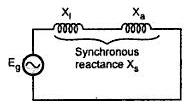Single Phase Short Circuit

Consider a single phase alternator operating under no load condition. This alternator is suddenly short circuited. As discussed earlier during initial moment of short circuit only leakage reactance of the machine limits the short circuit current. Under steady state, the armature reaction produces a demagnetizing flux which we take as synchronous reactance. Let the resistance of armature winding be small and can be neglected.
Immediately after the short circuit, the D.C. offset currents appear in the armature winding which can be computed separately on an empirical basis. Thus symmetrical short circuit currents are to considered only. Due to theorem of constant flux linkages, the air gap flux cannot change instantaneously for counterbalancing the demagnetizing effect of armature circuit current, the current is initially limited by leakage reactance only. The currents are thus induced in the field winding and the damper winding in a direction to help the main flux. Thus reactances Xf,  Xd are in parallel with Xa during initial period. The equivalent circuit is shown in the Fig. 1. and the equivalent reactance in this case is called subtransient reactance.

These currents appearing in the damper winding and the field winding decay depending upon winding time constants The damper winding has inductance less than that of field winding and hence current in it dies out first and afterwords Xd is said to be effectively open circuited. The machine reactance changes from its value of subtransient to transient consisting of parallel combination of Xf and Xad. This is shown in the Fig. 2.
The current in the field winding also dies out and we say that the machine is operating in the combination of Xf   and Xa. The equivalent circuit at steady state is shown in the Fig. 3.Fig. 3

The subtransient and transient reactances are respectively given by,

It can be seen that X'' X' X. Thus the machine offers variable reactance. As X'' is smallest initially current is very large which is reduced subsequently when currents in damper winding and field winding die out.
The currents are given by,

The oscillogram of current neglecting d.c. offset currents is shown in the Fig. 4.Fig. 4